# ML Wiki

## Residuals

Residuals is the difference between actual values and predicted values

$i$th residual is:

• $e_i = y_i - (b_0 + b_1 x_i) = y_i - b_0 - b_1 x_1$

Mean of $e$:

• $\bar{e} = \bar{y} - b_0 - b_1 \bar{x} = \bar{y} - (\bar{y} - b_1 \bar{x}) - b_1 \bar{x} = 0$

What about $\text{Var}(e)$?

• $e_i = y_i - b_0 - b_1 x_i = y_i - (\bar{y} - b_1 \bar{x}) - b_1 x_i = (y_i - \bar{y}) - b_i (x_i - \bar{x}) = (y_i - \bar{y}) - R \cfrac{s_y}{s_x}(x_i - \bar{x})$
• so,
$(e_i - \bar{e})^2 = (y_i - \bar{y})^2 + (R \cfrac{s_y}{s_x})^2 (x_i - \bar{x})^2 - 2 (\bar{y} - b_1 \bar{x}) \cdot R \cfrac{s_y}{s_x}(x_i - \bar{x})$
• $\text{Var}(e) = \cfrac{1}{n - 1} \sum (e_i - \bar{e})^2 = s_y^2 + (R \cfrac{s_y}{s_x})^2 s_x^2 - 2R \cfrac{s_y}{s_x} \cfrac{1}{n - 1} \sum (x_0 - \bar{x})(y_i - \bar{y}) =$ (note the orrelation coefficient again!)
• $= s_y^2 + R^2 s_y^2 - 2R\cfrac{s_y}{s_x} s_x s_y R = s_y^2 + R^2 s_y^2 - 2R^2 s_y^2 = s_y^2 (1 - R^2)$

So

$\text{Var}(e) = \text{Var}(y)(1 - R^2)$

## Coefficient of Determination

The regression multiplies the variance of $y$ by $(1 - R^2)$

• Or, the regression line removes (or reduces) a fraction of $R^2$ of the variance of $y$
• Or we say it "explains a fraction of $R^2$ of the variation"

$R^2$ is called coefficient of determination - and says what fraction of $\text{Var}(Y)$ has been explained by the linear relationship

Examples:

• $R^2 = 0$: the linear relationship explains nothing (so no linear relationship between $X$ and $Y$)
• $R^2 = 1$: the linear relationship explains everything - no left-overs, no uncertainty
• $R^2 = 0.0186$: only 1.86% of variation was explained by the linear model - so there hardly is a linear relation. The rest of the variance (98%) is due to something else

Let's take a look at the example again:

•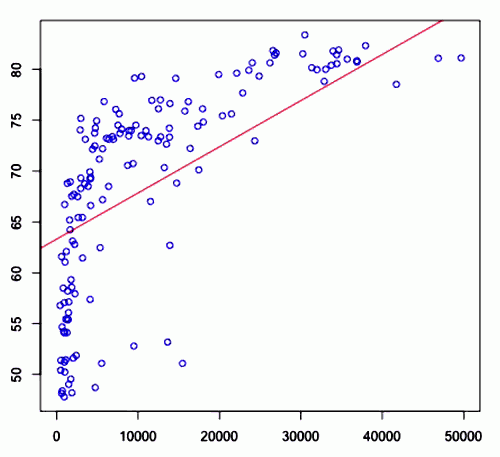• $R^2$ = 0.4033
• so it means quite a bit of variance there is explained by linear model
• but still it doesn't explain everything - indeed the real data doesn't seem to have linear relationship

## Residual Analysis

Are there any other kinds of relationships between $X$ and $Y$, not captured by regression?

### Ideal case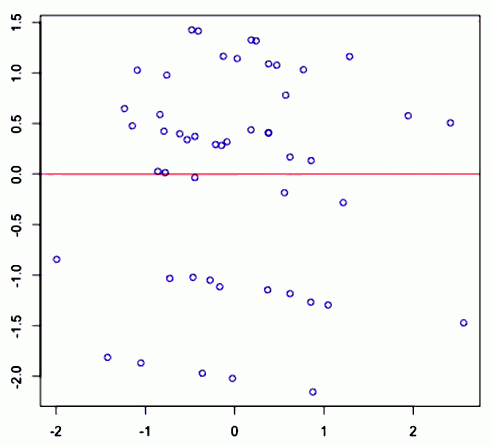• This is a good case because after taking out linear relationship there's no particular pattern in residuals: only independent errors are left
• So overall there's no particular trend and that means that the regression really tells us something about the relationships between $X$ and $Y$

### Another Example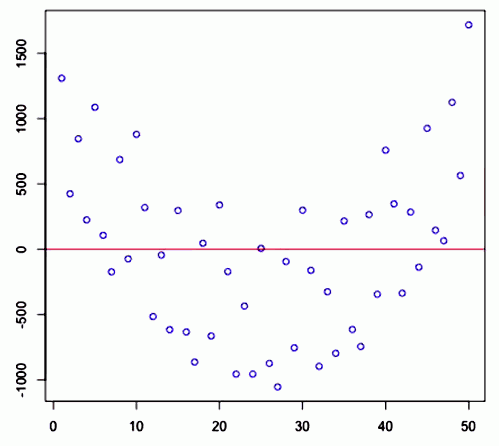And the same here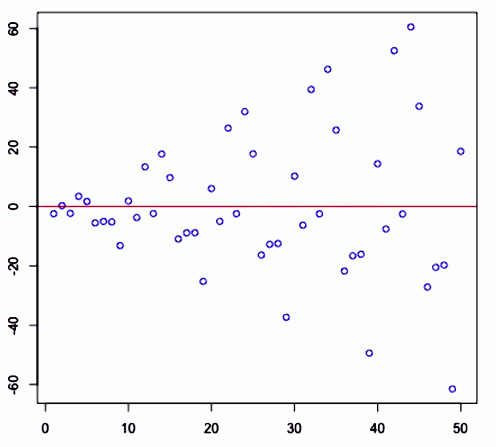In both cases the linear relationship doesn't describe the whole story and we see there are apparent patterns in the residuals in both cases

## Logarithmic Transformation

• To improve the situation we could try to transform the variables before applying regression.
• Most common transformation is logarithmic

So we have the following: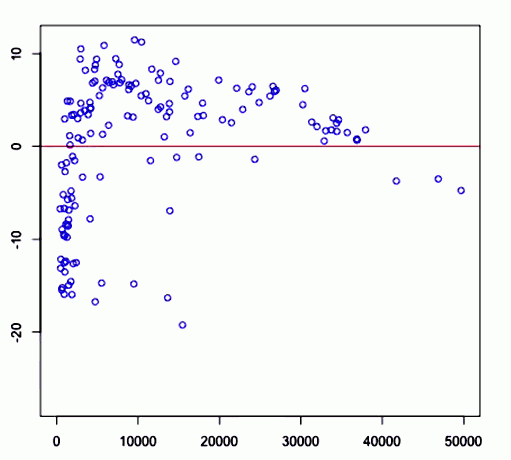Recall that in this case $R^2 = 0.40$

If we calculate $\log_{10} x$ what we get is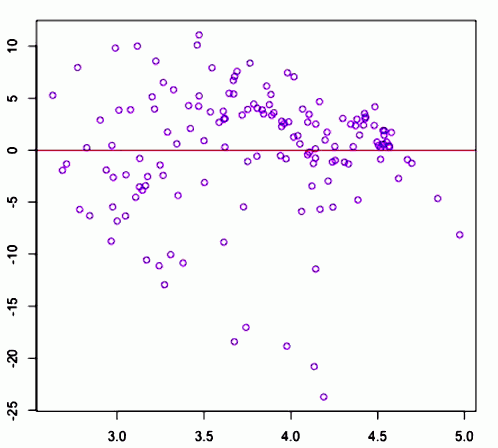Now we're able to fit a better regression line and in this case $R^2 = 0.6576$

Here we interpret a slope of 14.93 as

• if $\log_b x$ increases by $1$, $y$ increases by 14.93
• or if $x$ is multiplied by $b$, $y$ increases by 14.93